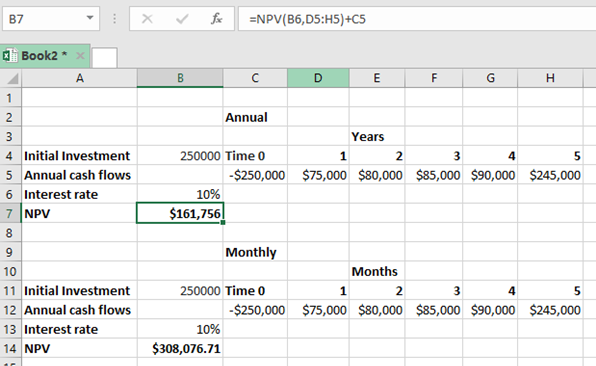Get instant live expert help with Excel or Google Sheets“My Excelchat expert helped me in less than 20 minutes, saving me what would have been 5 hours of work!”

#### Post your problem and you’ll get expert help in seconds.

Your message must be at least 40 characters
Our professional experts are available now. Your privacy is guaranteed.

# The Difference Between Annual vs. Monthly NPV in Excel

While working to find the NPV of a given data in an excel spreadsheet, excel takes annual assumptions. This might not always be the case, given that there are times when we calculate NPV based on a monthly cash flow. This calls for us to understand the difference between annual vs monthly NV in excel, as explained in this post.Figure 1: Difference between annual vs monthly NPV in excel

The calculation of the NPV based on an annual interest rate is a straightforward venture, given that the excel function is set to anticipate the rate as annual. But to get the returns based on a monthly cash flow, we have to set the rate to reflect the monthly status.

## General syntax of the annual NPV

To get the annual net present value, we need to use the following formula;

`=NPV(rate, range of projected value) + Initial investment`

## General syntax of the monthly NPV

`=NPV(rate/12, range of projected value) + Initial investment`

Note that the only difference comes in where we have the rate.

If we do not divide the rate by 12 months, then the cash flows will be discounted too aggressively by the excel function thinking that each column represents a year, and not a month.

In the above example, we have the first part showing the NPV for the annual rate, the second part shows the NOV for the monthly rate.

Notice that unlike in the first part where we have used the rate as it is (10%), we have divided it by 12 months in the second part, (10%/12). This is necessary if we want to reflect the monthly status of the cash flows.

## Instant Connection to an Expert through our Excelchat Service

Most of the time, the problem you will need to solve will be more complex than a simple application of a formula or function. If you want to save hours of research and frustration, try our live Excelchat service! Our Excel Experts are available 24/7 to answer any Excel question you may have. We guarantee a connection within 30 seconds and a customized solution within 20 minutes.

Solution examplesI have a figure which is the sum of 9 months of payments. Each payment decreases by the same amount (X) so the first payment is Y, second payment is Y-X, third is Y-(Xx2), forth is Y-(Xx3) and so on. I am trying to calculate what each of the 9 payments should be.
Solved by A. W. in 60 mins